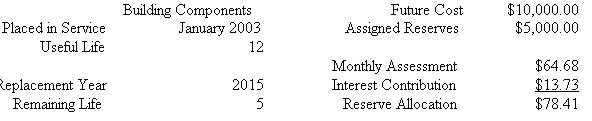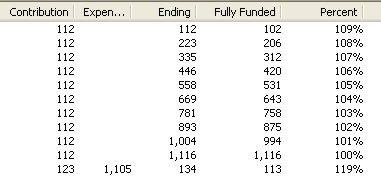Of course, a reserve study is about planning to have sufficient funds for future ‘events’. A discussion about philosophies of the study will be left for another time. Most studies are done by pooling all future expenses. Distribution of funds on a per component bases is often neglected because it really does not lend to what the association considers adequately funded..

But funding at the component level is often presented in a study even when some other funding philosophy is adopted. This is an explanation of component funding.

Consider a simple case where you plan to make a purchase in the future. You have some fraction of the funds needed in an account today so will have to make regular contributions to this account to meet the future purchase. If the account is not interest bearing the contributions are pretty simple to calculate. But most accounts are interest bearing and this interest can have a considerable effect on the needed contribution. In Reserve Analyst we use a financial math engine that calculates the numbers. You can find a description of the math by searching annuity and annuity due on the web.

Example.

Monthly Payment: 64.68

Fully Funded

This is an old definition with a simple formula. Take the present replacement value ‘times’ the age of the component ‘divided by’. the useful life.

Fully Funded = present value * age / useful life.

As you can see this is just an approximation of required funds when interest on reserves and inflation of the component, (future cost), should be considered. There are more complicated formulas that take inflation and interest into account but this is done only to get closer to a true annuity calculation.

Distribution of funds

At the beginning of a study there is usually money on deposit. So just as in the simple case above, needed contributions should take into account money already available for expenses. So these funds are distributed among the components at the start of calculations. First is to distribute starting with the earliest replacements the fully funded amount. If there are funds left after going through the components next is to distribute to the present cost of replacement. If funds are still available after this second pass, next is to distribute to twice the cost of components that will be replace in the first years. Any funds left are considered excess funds.

Now a calculation of required contribution can be done for the components. This snip from a test report and reflects the payment example above.Also shown are the interest earned in the first month and a sum called ‘Reserve Allocation’.

These numbers are summed and presented in the detail summary as ‘Total of All Assets’.

Contingency

An association may decide to keep a percentage of Component Method funds aside. This contingency is taken from the deposited funds before distribution is done. After the required contribution to funds is calculated it is increased by this percentage in each year of the projection.

CFM Velocity

This is provided so the preparer can modify the funding of components to create a pleasing look to the projection. Here is a one component projection with only inflation set at 3%The calculation for the contribution is annuity due on future cost while the calculation for fully funded is based on the current cost in each year.